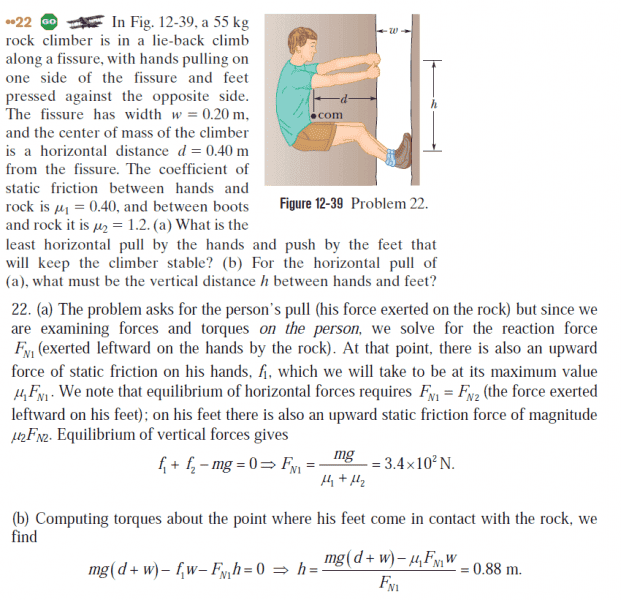# Static Equilibrium

## Homework Statement

I have a question about the following question:Firstly, shouldn't there be a normal force for each hand (part a), but here they treat it as one normal force.

Also in part b, why isn't the torque for Fn1h = 0? Since that is the pivot point, isn't the moment arm zero?

Simon Bridge
Homework Helper
Firstly, shouldn't there be a normal force for each hand (part a), but here they treat it as one normal force.
How is the result different if you give each hand a separate normal force?

Also in part b, why isn't the torque for Fn1h = 0? Since that is the pivot point, isn't the moment arm zero?
##F_{N1} \neq 0## and ##h\neq 0## therefore ##F_{N1}h \neq 0## ... take a closer look: what are they using for the pivot point in that calculation?

Wow. The pivot point is the location of the foot and N1 is for the hands. I see. Thank you.

Simon Bridge